Type a math problemalgebra
trigonometry
statistics
calculus
matrices
variables
list
AC
log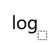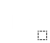ln
(
)
7
8
9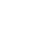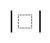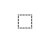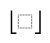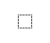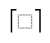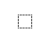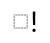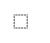τ
π
4
5
6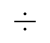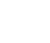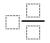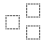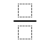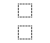%
θ
1
2
3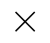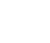<
>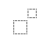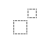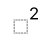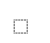x
i
0
.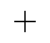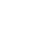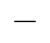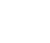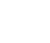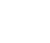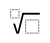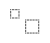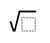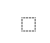y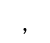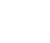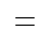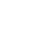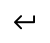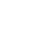Solve for xSteps for Solving Linear Equation
Swap sides so that all variable terms are on the left hand side.
Subtract from both sides.
Subtract from to get .
Divide both sides by .
Divide by to get .View solution steps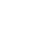Graph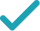Graph Both Sides in 2D
Graph in 2D
Powered by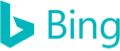2x+3=5
Swap sides so that all variable terms are on the left hand side.
2x=5-3
Subtract 3 from both sides.
2x=2
Subtract 3 from 5 to get 2.
x=\frac{2}{2}
Divide both sides by 2.
x=1
Divide 2 by 2 to get 1.